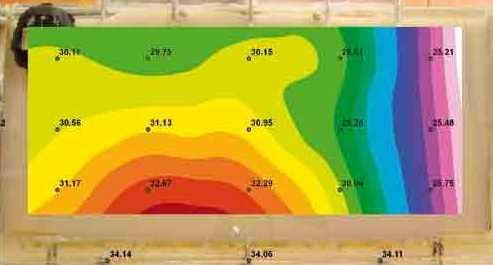# Examining a Coupled Continuum Pipe-Flow Model for Groundwater Flow and Solute Transport in a Karst aquifer

• Bill X. Hu Department of Earth, Ocean and Atmospheric Sciences, Florida State University, Tallahassee, FL 32306

### Abstract

A coupled continuum pipe-flow (CCPF) model has been de­veloped for groundwater flow and solute transport in a karst aquifer withconduits. Groundwater flow in conduits is simu­lated througha pipe flow model and flow in fissured matrix rock is described by Darcy’s law. Water mass exchange between the two domains is modeled by a first-order exchange rate method. In this study, we investigate mathematical well-posed­ness (mathematical term, whichmeans solution existence and uniqueness) of the CCPF model, develop a finite elementary method to numerically approximate the mathematical model and study the convergence of the numerical method. The study results prove the modeling approachis mathematically well posed and numerically converged. To study the accuracy of the CCPF model, a recently developed Stokes-Darcy (SD) model and CCPF model are compared withlaboratory experimental results. It was found that the SD model simulations matchwell withexperimental results, but the CCPF model overestimates the hydraulic head in the matrix, especially around the matrix and conduit interface. The model underestimates solute trans­port in the conduit and does not capture the plume distribution in the matrix. In comparison withthe SD model, the CCPF model requires an additional parameter, the first-order mass exchange rate, and the parameter is normally obtained throughinverse method curve fitting. The SD method may provide an approachto directly estimate the parameter value.